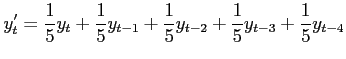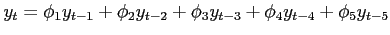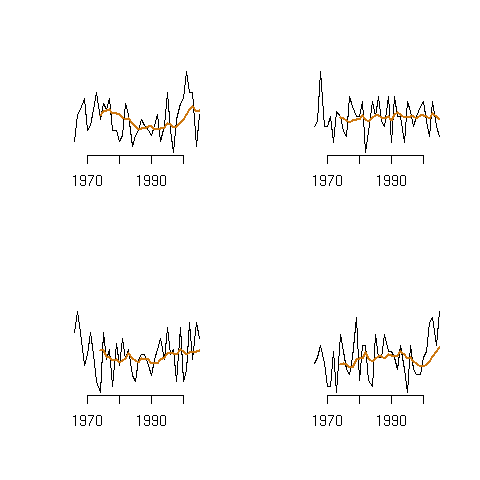Statistics

# Hurricanes have not increased: misuse of running means

Most statistics purporting to show that there has been an increase in hurricanes do not use the best statistical methods. I want to highlight one particular method that is often misused, and which can lead one to falsely conclude that trends (increasing or decreasing) are present when they actually are not. Read my original post to learn more about this.

That technique is the running mean. As you can see in the rather dramatic graphic from Science Daily, a 9-year running mean has been plotted over the actual hurricane numbers (up to 2005 only) in the North Atlantic. It looks like, in later years, a dramatic upswing is taking place, doesn’t it? This type of plot has shown up in many scientific, peer-reviewed papers.#### Don’t be turned off by the equations! Something very surprising is coming at the end of this article and you will be rewarded if you read to the end.

What is a running mean? A p-year running mean converts a series of actual, observed numbers into a statistical estimate, or model, of what a supposed underlying trend of those numbers might actually be. Because it is a model, its use must first be justified. In math, a 5-year running mean looks likewhere the symbol y indicates the hurricane numbers, and the subscripts t, t-1 and so on indicate the time period: time t is now, time t-1 was last year (now minus one year) and so on. The superscript on the symbol y to the left of the equal sign indicates that this is the modified data value plotted, and is not the actual number. Even if you’re afraid of math, this equation should be fairly easy to understand: the current, modified, number is just the mean of the last 4 observations and the most current one.

Additionally, in the mathematics of time series models, an auto-regressive series of order 5 is written like thiswhich shows how the current data point is predicted by a weighted sum of past values, and where the weights are the coefficients ?. Just let all the ? = 1/5 and you have a similar running mean structure like that above. The point is this: using a running mean implies an underlying statistical time series model. Which is OK, as long as the data support such a model.

Do they for hurricanes? No.

In order to justify using auto-regressive time series models, you start by looking at something called an auto-correlation plot, which is a plot of how each year’s number of hurricanes is correlated with the previous year’s number, and how this year’s number of hurricanes is correlated with the number of hurricane from two years ago and so on: the number of previous years is called the lag. If any of these correlation lags are significant, then you can use an auto-regressive time series model for this data. If none of these correlations are significant, then you cannot.

Here is a picture of the auto-correlation of hurricane number (number of storms s) for the North Atlantic using data from 1966 to 2006.None of the correlations reach above the horizontal dashed lines, which means that none are significant, and so a simple running mean should not be used to represent North Atlantic hurricane numbers.

So far, so good, right? Now let’s look at some made up, fictional data. Take a look at the following pictures, which are all simulated hurricane numbers; one of them looks pretty close to what the real data looks like. The running-mean even shows a healthy upward trend, no doubt due to global warming. But what do these pictures really show?To get this data (the R code to make it yourself is pasted bellow), I simulated hurricane numbers (Poisson with mean 10) for pretend years 1966 to 2005, four separate times. Each year’s number is absolutely independent of each other year: to emphasize, these are totally random numbers with no relationship through time. I also over-plotted a 9-year running mean (red line). Because all of these numbers are independent of one another, what we should see is a flat line (with a mean of 10). The reason we do not is because of natural variation.

I only had to run this simulation once, but pay attention to the lower-right hand numbers, I got something that looks like the actual North Atlantic hurricane numbers. The 9-year running mean is over-emphasizing, to the eye, a trend that is not there! Actually, this happens to two of the other simulated series. Only one shows what would expect: a (sort of) straight line.

Like I said, I am including the code I used to make these plots so that, if you are curious, you will see how exceptionally easy this is to do.

Good statistical models are hard to do. See some of these posts for more discussion and to find some papers to download.

R code to make the plots. You must first have installed the package gregmisc.

```library(gregmisc)
par(mfrow=c(2,2))
for (i in 1:4){
x=rpois(40,10)
plot(1966:2005,x,type='l',xlab="",ylab="",axes=F)
axis(1)
1.TC says: